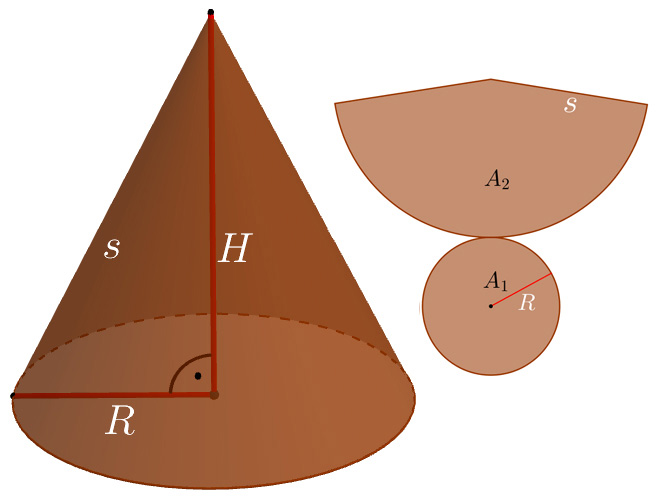# Cone## Area

$A={A}_{1}+{A}_{2}={R}^{2}\pi +R\pi s$

## Base Area

${A}_{1}={R}^{2}\pi$

## Side Area

${A}_{2}=R\pi s$

## Volume

$V=\frac{{R}^{2}\pi H}{3}$
Keywords: cone, area, volume